# Gradients of Lines (Positive or Negative)

In this worksheet, students identify lines with positive, negative, zero and infinite gradients.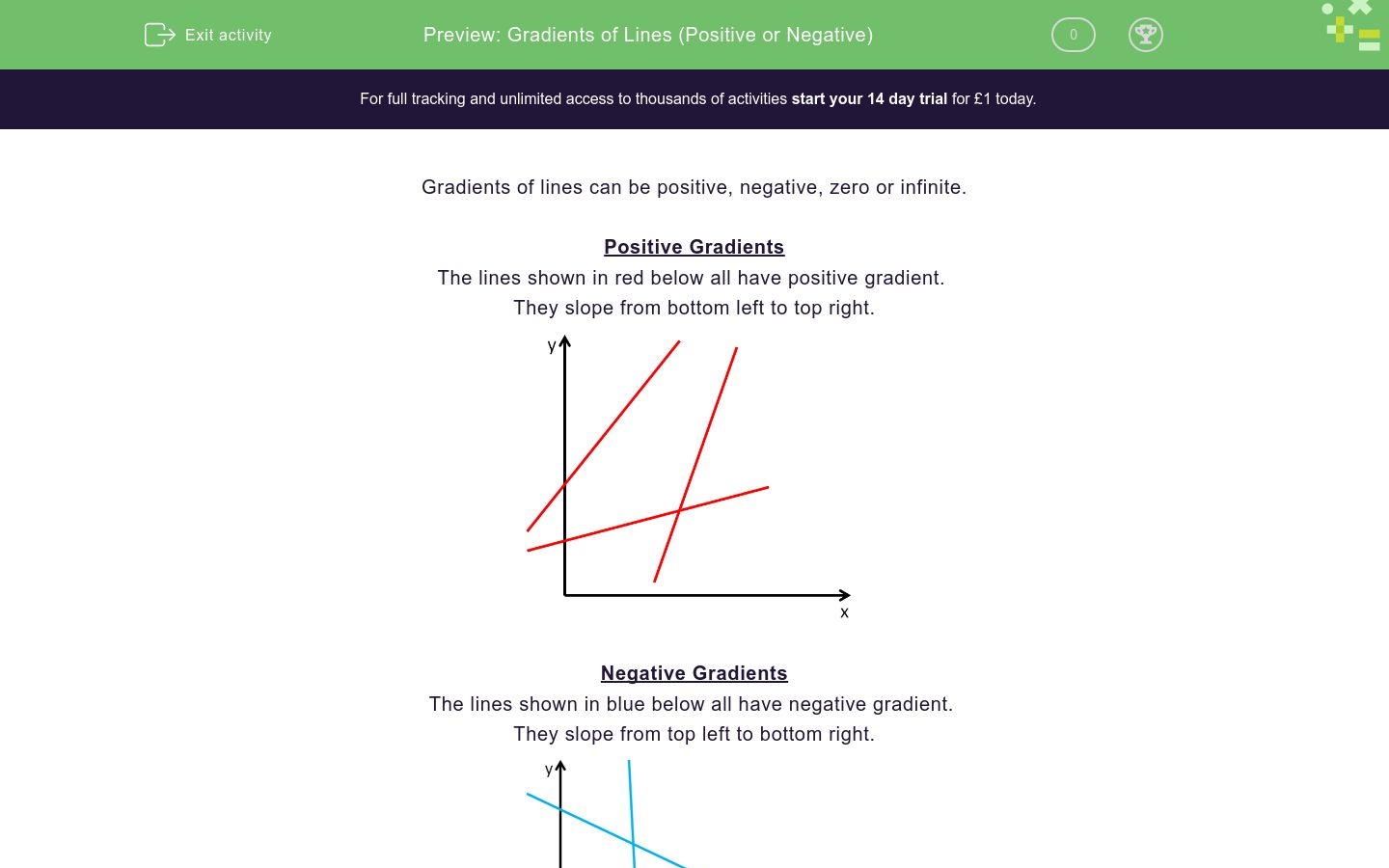Key stage:  KS 4

Curriculum topic:  Algebra

Curriculum subtopic:  Use y = mx + c to Identify Parallel and Perpendicular Lines

Difficulty level:### QUESTION 1 of 10

Gradients of lines can be positive, negative, zero or infinite.

The lines shown in red below all have positive gradient.

They slope from bottom left to top right.The lines shown in blue below all have negative gradient.

They slope from top left to bottom right.The lines shown in green below all have zero gradient.

They lie flat or horizontal and are parallel to the x-axis.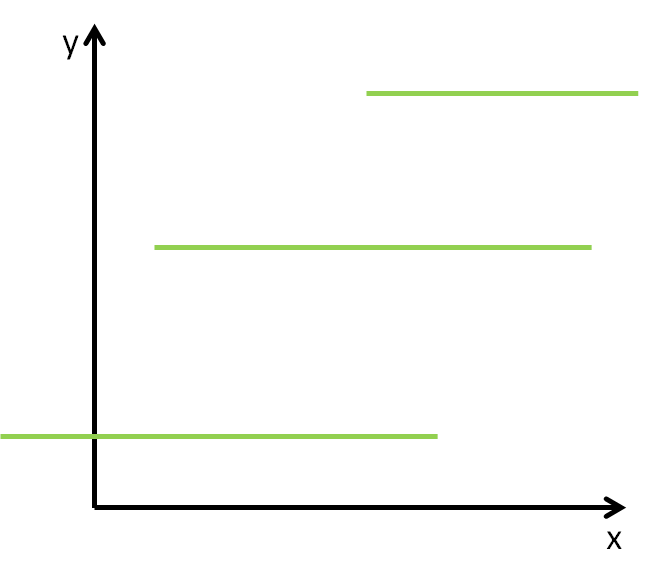.

The lines shown in orange below all have infinite gradient.

They are vertical and are parallel to the y-axis.Look at the red line and state whether its gradient is positive, negative, zero or infinity.Positive

Negative

Zero

Infinity

Look at the red line and state whether its gradient is positive, negative, zero or infinity.Positive

Negative

Zero

Infinity

Look at the red line and state whether its gradient is positive, negative, zero or infinity.Positive

Negative

Zero

Infinity

Look at the red line and state whether its gradient is positive, negative, zero or infinity.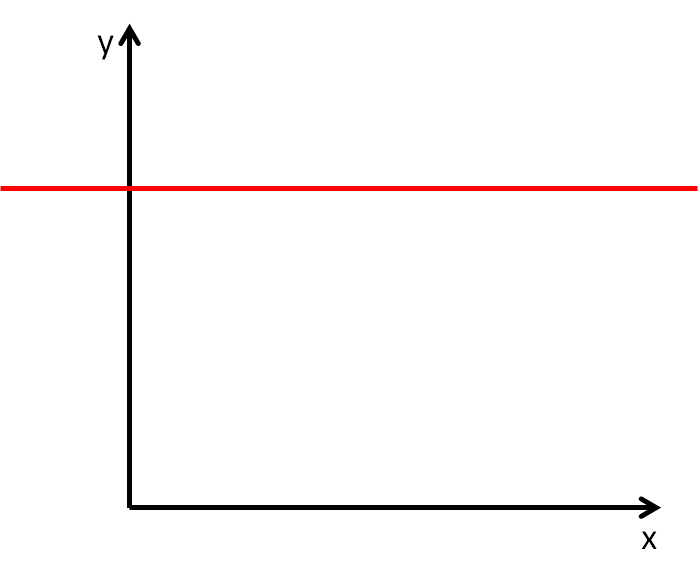Positive

Negative

Zero

Infinity

Look at the red line and state whether its gradient is positive, negative, zero or infinity.Positive

Negative

Zero

Infinity

Look at the red line and state whether its gradient is positive, negative, zero or infinity.Positive

Negative

Zero

Infinity

Look at the red line and state whether its gradient is positive, negative, zero or infinity.Positive

Negative

Zero

Infinity

Look at the red line and state whether its gradient is positive, negative, zero or infinity.Positive

Negative

Zero

Infinity

Look at the red line and state whether its gradient is positive, negative, zero or infinity.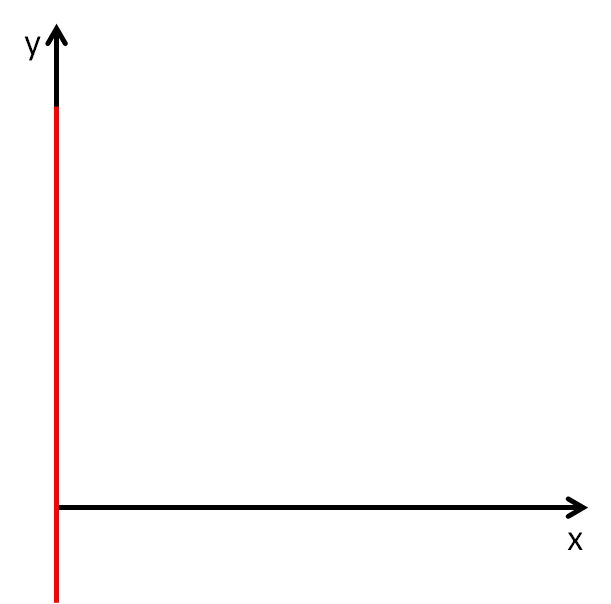Positive

Negative

Zero

Infinity

Look at the red line and state whether its gradient is positive, negative, zero or infinity.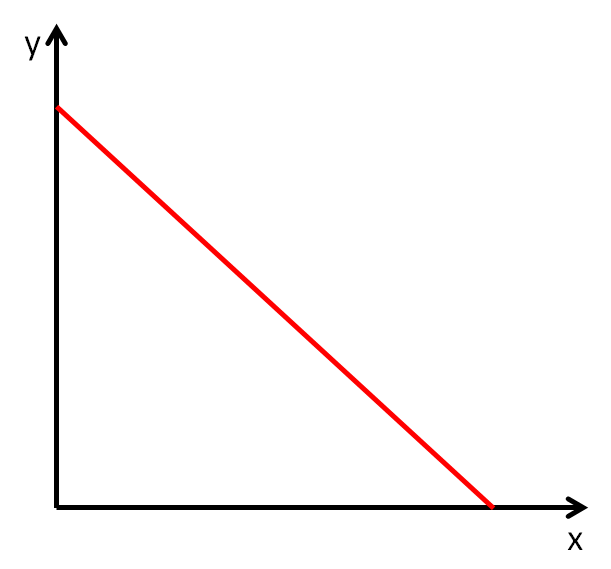Positive

Negative

Zero

Infinity

• Question 1

Look at the red line and state whether its gradient is positive, negative, zero or infinity.Positive
• Question 2

Look at the red line and state whether its gradient is positive, negative, zero or infinity.Positive
• Question 3

Look at the red line and state whether its gradient is positive, negative, zero or infinity.Negative
• Question 4

Look at the red line and state whether its gradient is positive, negative, zero or infinity.Zero
• Question 5

Look at the red line and state whether its gradient is positive, negative, zero or infinity.Negative
• Question 6

Look at the red line and state whether its gradient is positive, negative, zero or infinity.Positive
• Question 7

Look at the red line and state whether its gradient is positive, negative, zero or infinity.Positive
• Question 8

Look at the red line and state whether its gradient is positive, negative, zero or infinity.Infinity
• Question 9

Look at the red line and state whether its gradient is positive, negative, zero or infinity.Infinity
• Question 10

Look at the red line and state whether its gradient is positive, negative, zero or infinity.